All news

# Modeling Physics with the Wolfram Language

Here’s another guest post from Allison Taylor at Wolfram Research. Today we’re looking at how to build simple physics models using the Wolfram Language.

If you’ve taken any introductory physics course, you’ve learned about Newtonian mechanics—conservation of energy and momentum, friction, harmonic motion, and so on. Idealized, classical motion can be broken down into a series of simple equations based on position, acceleration, and velocity. To derive these equations requires solving differential equations—something the Wolfram Language does very well with a function called NDSolve[].

For example, to model basic freefall, we just need to determine our initial conditions—acceleration y” is given by gravity, 9.81 m/s²; initial position is 150 m; and initial velocity y’ is 0. From these conditions, NDSolve[] can figure out the motion for a given range.

`freefall=NDSolve[{y''[t]==-9.81,y==150,y'==0},y[t],{t,0,5}];`

`Plot[y[t]/.%,{t,0,5}]`

produces: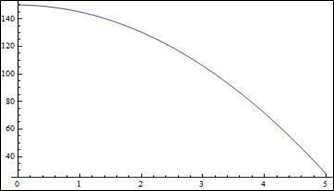So, in the above case, if I dropped a ball from a height of 150m, it would take approximately 5 seconds to reach the ground. Simple! But we can take this a step further with a function called WhenEvent[]. If I were to try to model a bouncing ball by hand, for example, I’d have to reevaluate the equations of motion every time the ball reached an “event” or change in conditions. The ball is in freefall from the air to the ground—but I can’t rely on those same values to model the ball bouncing from the ground back into the air.

Using WhenEvent[] inside NDSolve[] lets you specify what happens when y[t]=0, or when the ball hits the ground. For this example, let’s say that when the ball hits the ground, it will reverse direction at 95% of its original speed. Now, every time the ball hits the ground, its velocity magnitude will continue to decrease by 5%. All inside one NDSolve[]—no more additional work for us!

```NDSolve[{y''[t]==-9.81,y==5,y'==0,    WhenEvent[y[t]==0,y'[t]->-0.95 y'[t]]},y[t],{t,0,10}];```

`Plot[y[t]/.%,{t,0,10}]`

produces: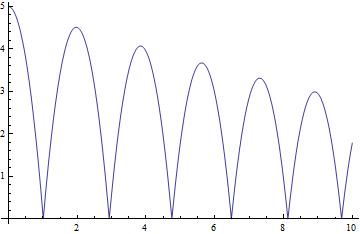Having these values automatically computed means we can also easily observe how energy changes over time. Take the values of position and velocity that are calculated and plug them into the classical equations for kinetic and potential energy:

```ball=NDSolve[{y''[t]==-9.81,y==13.5,y'==0,    WhenEvent[y[t]==0,y'[t]->-0.95 y'[t]]},{y[t],y'[t]},{t,0,10}];```

`kin[v_]:=.5 v^2;`

`pot[y_]:=9.8 y;`

`energy[y_,v_]:=kin[v]+pot[y];`

```GraphicsGrid[{{Plot[y[t]/.ball,{t,0,10},AxesLabel->{"t","y"}],    Plot[Evaluate[{kin[y'[t]],pot[y[t]],energy[y[t],y'[t]]}/.ball],{t,0,10},    Filling->{3->0},AxesLabel->{"t","energy"}]}}]```

produces: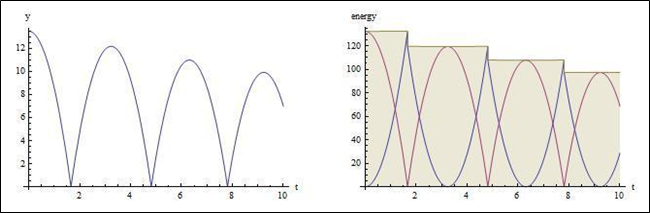The graph on the left shows the ball’s position, and the graph on the right shows the potential energy in red, kinetic energy in blue, and total energy in brown. Notice that the total energy decreases stepwise with each energy-dissipating bounce.

And further! What if the ball was not bouncing on even ground? What if, instead, the ball was bouncing down a flight of stairs? The same principles are used, with an additional a[t] specifying how many steps of width one the ball must drop down. When the ball reaches the end of the stairs, “RemoveEvent” is used to stop the condition from continuing to be in effect.

```steps=NDSolve[{x''[t]==0,y''[t]==-9.8,y==6,y'==0,x==0,x'==1,a==5,    WhenEvent[Mod[x[t],1]==0,If[a[t]>0,a[t]->a[t]-1,"RemoveEvent"]],    WhenEvent[y[t]==a[t],{{x'[t],y'[t]}->.9 {x'[t],-y'[t]}}]},{x[t],y[t],a[t]},    {t,0,15},DiscreteVariables->{a[t]}];```

```Show[ParametricPlot[Evaluate[{{x[t],y[t]},{x[t],a[t]}}/.steps],{t,0,15},    ImageSize->300],Plot[{0,Floor[6-x]},{x,-1,15},PlotStyle->Thick,    Exclusions->None],Frame->{{True,False},{True,False}},    FrameLabel->{"x","y"}]```

produces: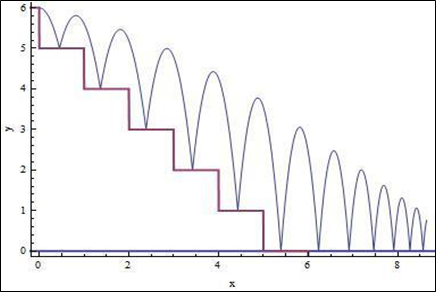And again, we can also model the energy alongside it, and have the Wolfram Language punch up the graphics using Reap[] and Sow[] to obtain the coordinates of the ball at each bounce.

```{ballsteps,{points}}=NDSolve[{x''[t]==0,y''[t]==-9.8,y==6,y'==0,    x==0,x'==1,a==5,WhenEvent[Mod[x[t],1]==0,If[a[t]>0,a[t]->a[t]-1,    "RemoveEvent"]],WhenEvent[y[t]==a[t],{{x'[t],y'[t]}->.9 {x'[t],-y'[t]},    Sow[{x[t],y[t]}]}]},{x[t],y[t],x'[t],y'[t],a[t]},{t,0,15},    DiscreteVariables->{a[t]}]//Reap;```

```GraphicsGrid[{{Show[ParametricPlot[Evaluate[{{x[t],y[t]},{x[t],a[t]}}/.ballsteps],    {t,0,15},ImageSize->300,Epilog->{Red,PointSize[Medium],Point[points]}],    Plot[{0,Floor[6-x]},{x,-1,15},Filling->{2->0},Exclusions->None],    Frame->{{True,False},{True,False}},FrameLabel->{"x","y"}],    Plot[Evaluate[{kin[y'[t]],pot[y[t]],energy[y[t],Norm[{x'[t],y'[t]}]]}/.ballsteps],    {t,0,15},Filling->{3->0},Frame->{{True,False},{True,False}},    FrameLabel->{"t","energy"},Ticks->None]}}]```

produces: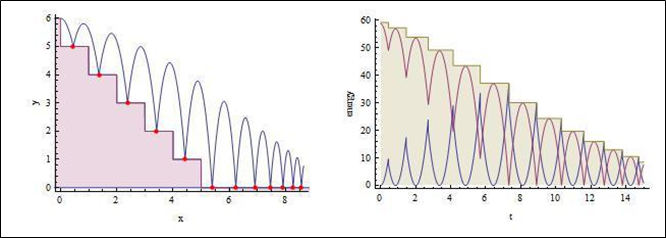Event[] inside NDSolve[] can be used to model all sorts of processes—from friction models to signal processing, to even simulating the human heartbeat. It lets you visualize many complex environments in a simple, cohesive way. Try it out!

That’s amazing and also rather scary. I always hated differential equations. Glad to know that if I’m ever brave/foolhardy enough to need them, there’s a language that can do it for us. Thanks Wolfram :)

I remember talking to my friend Mark, who as doing the Maths Tripos, about some neat new tricks I’d learnt for finding analytic solutions to differential equations on the Natural Sciences Tripos. He looked at me pityingly and said something along the lines of “don’t be ridiculous, we just solve these things numerically these days”. Lesson learned: simulation and numerical methods are now a first-class component of the scientific method alongside theory and experimentation.

Wow! Examples like this are perfect for showing how programming is useful for cross-discipline teaching.

I’ve always found pure maths difficult since the lack of a real result means you can’t determine how sensible your answer is.

Applying this stuff to real applications will help a lot of kids/adults not only understand why it is useful but also see why that missing minus sign is so important…

Now who’s turn is it to write the GPU powered physics engine for the Raspberry Pi?

Reminds me of the Nuffield A Level Physics a few decades ago, which had the ‘Dynamic Modelling System’ running on BBC Bs. But MUCH more powerful.
One of my examples then was a cat swung in a circle, treated as a mass on an elastic spring. Sorry to all felines… it was a gedanken experiment!
Great addition to what Pi can offer.
(and Wolfram Alpha is pretty impressive too…)

So, two challenges methinks:
1.) Use Wolfram to generate ‘snapshot’ pictures (graphs, whatever) of, for example, the ‘position’ of the ball bouncing down the stairs – and then use the Pi to turn those snapshot sequences into a playable movie
2.) Simulate two identical pendulums hanging from a taut string, itself supported from to fixed points. Consider the pendulm length to be ‘unit=1’, and fixed. Then allow two variables – length of string between the pendulum attachment points, and the (equal/identical) lengths of string between the pendulum attachment points and the taut-string (catenary) end points. Model this, and allow movies (as above) to be created.

Why? Well, I sued to have a little model (two golf balls, each hanging from a taut string) to demonstrate the transfer of energy (due to resonance) between ‘tuned circuits’. I used to use this back in the early ’80s as an aid to understanding how the predecessor to GPS actually worked – or, at least, how we got the ground-based transmitter circuit to get oscillations into the antenna.

I’d be grateful for any attempted solutions – I don’t stand a chance, my (analytical) maths skills are just not up to it!! But, I’d be happy to ‘break down’ the Wolfram code, to try and figure out how some genius (you, dear reader??) has attacked the problem!

Cheers,
Niall

I would love to see the data fed into Pi3D for simulating, and also tied into a hardware setup.

Watch this space … space … space … space … ;)

It’s actually possible to export animations in the Wolfram Language! All you need to do is generate a table of the “snapshots” or frames you want to include, and Export[] will compile them into a .mov, .gif or a number of other file formats.

.. as long as you don’t drink-and-derive

*groan*

Not bad… now do it for a ‘Slinky’ on the same set of stairs…

Eli.

As great as the news that Wolfram stuff is no bundled with the Pi might sound at first, I am not enterly convinced bundling proprietary stuff like this with the Pi.
I know there are no on-par alternatives, but with enough need for it one would eventually emerge – maybe even written by someone owning a Pi who wished he/she had something compareable on it.

This way pupils/students get used to this proprietary product for free, and once they have to use it for their professional work they are basically already tied to this stuff.

I know the comparison is a bit fishy, but would you also bundle Microsoft Office in case Microsoft would decide to do a Linux port and give it away for free to every Pi owner?

Sorry, wrong place for a a OS vs commercial crusade…

Wolfram’s contribution to the Pi is fantastic and should be applauded.

When I was a former student, we also got almost everything you could imagine for free – but should I apploud those companies for that … I am in doubt.

I know quite a number of collagues who built stuff with that proprietary crap and found startups, when they suddenly found that had to pay a fortune to continue what they’d started at university.

I think I know that university! Is it the one where all the students are so utterly stupid that they don’t realise that software actually costs money? It was in the Daily Mail – evil companies took advantage of their stupidity by giving them free stuff and saying that it was free. And they fell for it!

But once they left for the real world they suddenly found out that they actually had to pay for this stuff! And it was a fortune, not like “Calculator” in Windows which is free. But it was too late: they were addicted in the brain by “proprietary crap” and all of their files locked in to proprietaryness and all their skills shaped by industry standard, world-class tools. Idiots.

Wolfram and Mathworks both provide low cost home versions of their software, so I see no problem here. At the end of the day programmers have mortgages and kids to feed, so not everything can be free. RPi even costs something, so I see no issues whatsoever with including this fantastic environment on the RPi. Especially if it can help people get to grips with important math concepts like calculus.

> Wolfram and Mathworks both provide low cost home versions of their software

As does Microsoft, but would the Foundation bundle MsOffice for free with the Pi? I seriously doubt that…

No, because MsOffice doesn’t work under Linux, and Windows doesn’t work on the Raspi.

Next question?

How do you conceive code like this? I get the gist, but when I actually try to reverse-engineer what is happening it takes forever to find out which “[” matches with which “]” and an expression that contains syntax such as: “]}}]},{” has to be considered unreadable. Do Mathematica coders actually write stuff like this in real time, or do they prototype smaller expressions that they then put together?